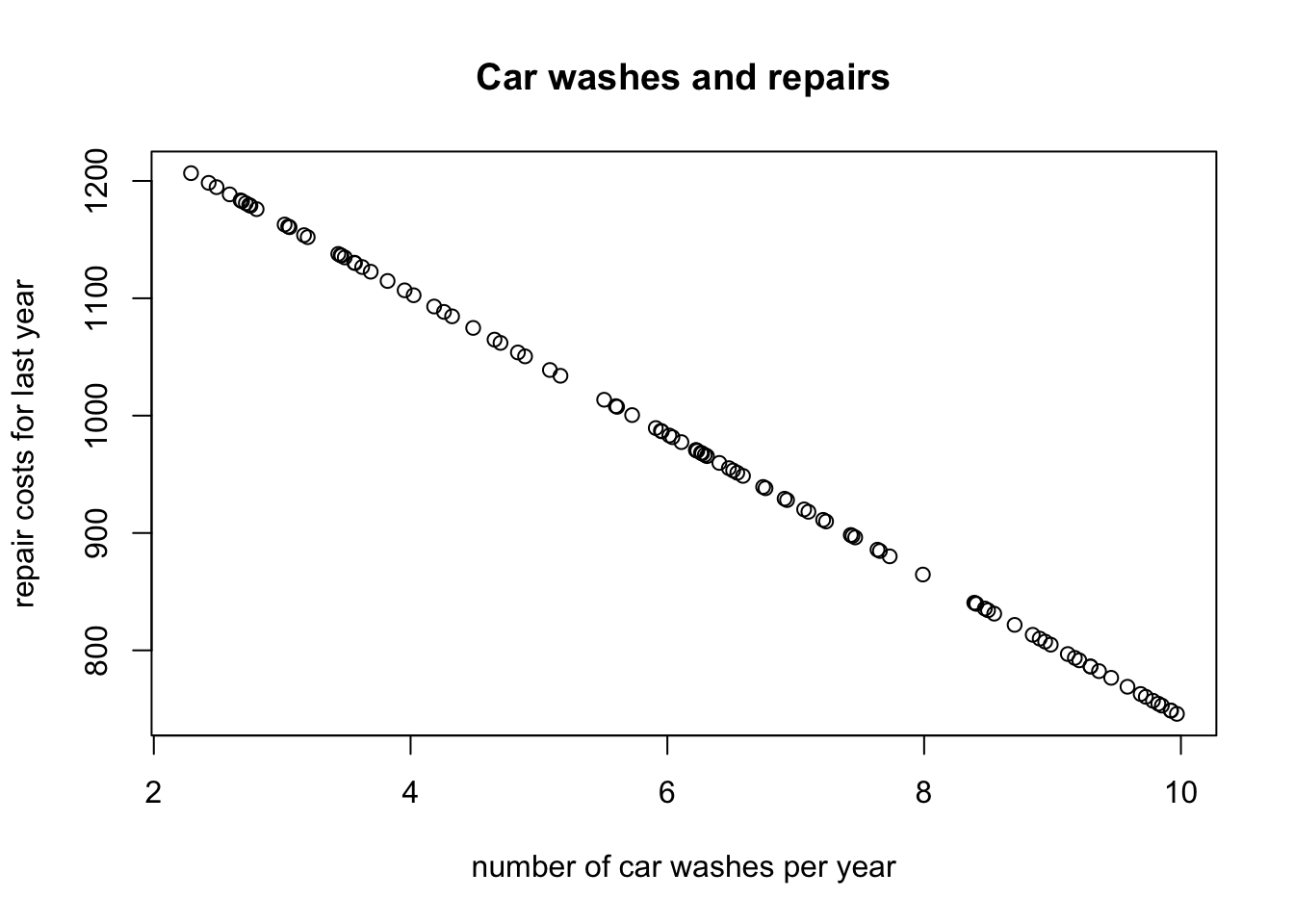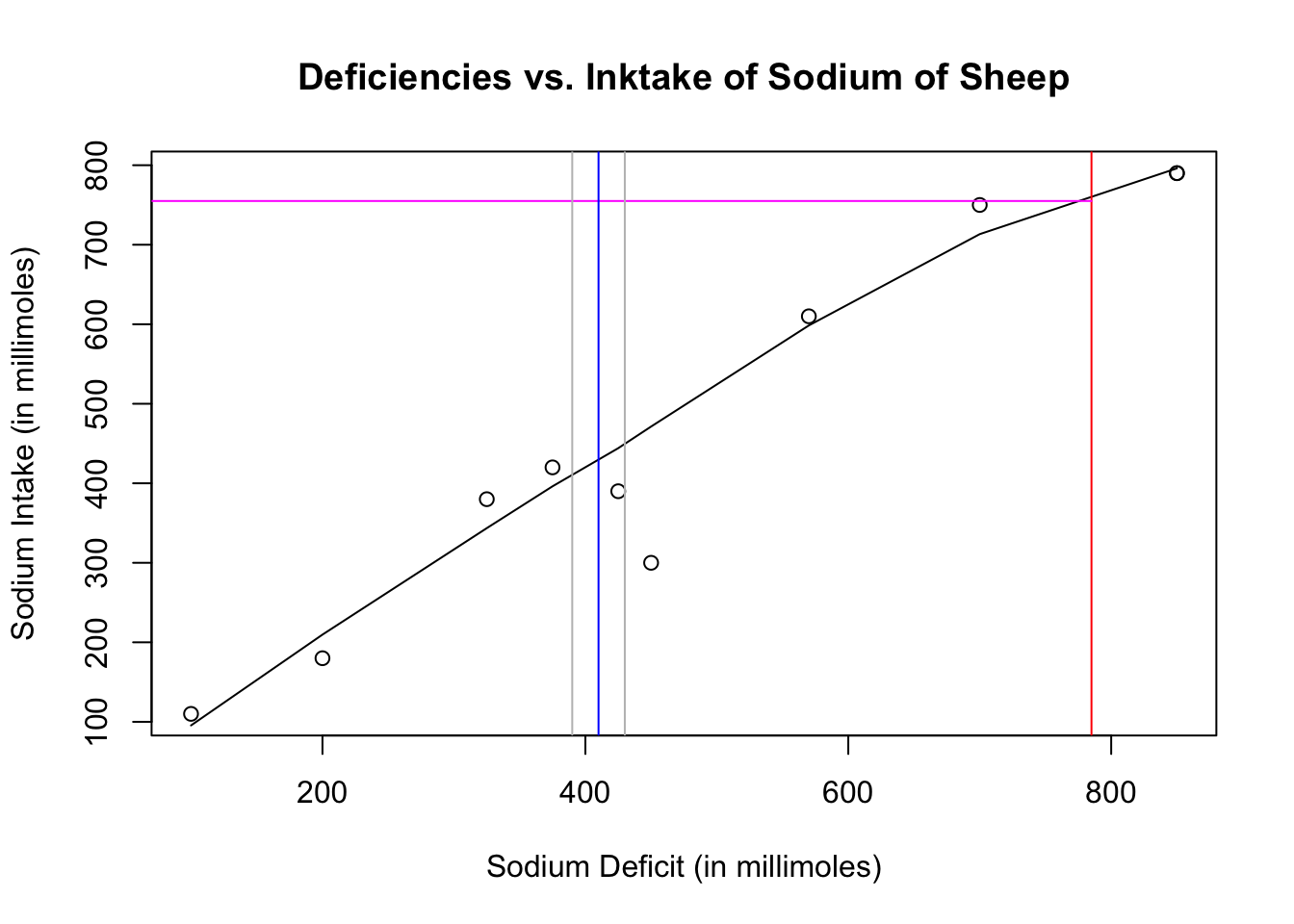Unit II Study Guide

## 1. Car washes and Repairs

Observations can be fairly simple and straightforward. Notice how the table gives the units for y. What do you think the units for x should be?

Mean Standard Deviation
x = number of car washes per year 6.4 3.78
y = repair costs for last year $955.30$323.50

The correlation for these two variables is r = -0.71.

### Questions you should be asking yourself during the test

• What does the negative number imply?
• How do you intepret a negative correlation number?
• What is the range of any correlation?
• Given knowledge of the range of the correlation, does r=-0.71 mean anything different to you?

### 1a. Find the equation of the least-squares regression lines

• What are the units of the equation of y?
• You have the equation, but start thinking about the sign of the slope and y-intercept. What does a negative slope imply?

### 1b. Interpret the slope of the least-squares line in context.

If you start thinking about what the data implies as you are writing out the equations, then you can start writing the “English” part of the exam.
Words like “in context” and “interpret” mean you should be writing sentances about your observations. Don’t forget units.

DRAW A PICTURE with meaningful units on the x and y axes. Dollars (\$) on the y, and “# of times washed / year” on the x.

The picture should reflect both the correlation (r=-0.71) and the slope (-60.7).## 2. Mass of Chicken Eggs

• Since the question was “eggs weighing more than 52 grams”, and 52 did not lie directly on the border of 1 exact standard deviation, you needed to use a z-score. Which means you need to scale the data down to a mean of 0, and a standard deviation of 1.

## 3. Linear relationship of Sodium deficiences and Sodium Intake

• Residual plots are needed to help you understand if your “straight line” model fits the data properly or not.
• Residual Plot?
• Context? The instructor is looking for an analysis in “English”!!

• 3b. Write the least-squares equation of this data.
• Include a definition of what x and y are. If you just write down the equation, you may not have proved you what x and y are. In this case, the deficit is the x or treatmeant (how much sodium was drained from the parotid gland of the sheep.) The y is the measured intake.
• 3c. How well does your model fit the data? Show statistical evidence.
• 3d. Estimate the amount of a sheep’s sodium intake if the sheep is found to be deficient in sodium by 785 millimoles.

deficit = c(100, 200, 570, 850, 700, 425, 375, 325, 450, 850)
intake =  c(110, 180, 610, 790, 750, 390, 420, 380, 300, 790)